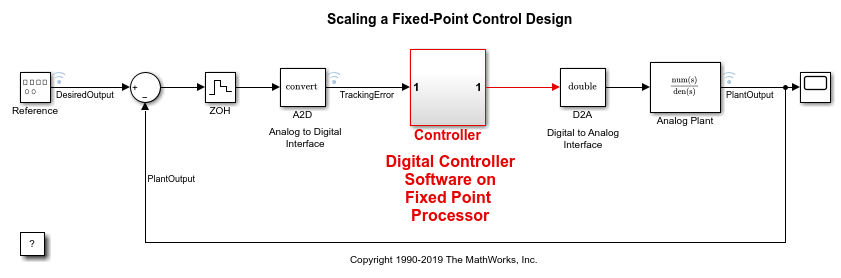# DataTypeWorkflow.Converter

Create fixed-point converter object

## Description

The `DataTypeWorkflow.Converter` object contains the object functions and parameters needed to collect simulation and derived data, propose and apply data types to the model, and analyze results. Use the `DataTypeWorkflow.Converter` object to perform the same fixed-point conversion tasks as the Fixed-Point Tool.

## Creation

### Syntax

``converter = DataTypeWorkflow.Converter(systemToScale)``
``converter = DataTypeWorkflow.Converter(referencedModelSystem,'TopModel',topModel)``

### Description

example

````converter = DataTypeWorkflow.Converter(systemToScale)` creates a converter object for the `systemToScale`.```
````converter = DataTypeWorkflow.Converter(referencedModelSystem,'TopModel',topModel)` creates a converter object with the specified referenced model, `referencedModelSystem`, as the system to scale.```

### Input Arguments

expand all

Name of the model or subsystem to scale, specified as a character vector.

Example: ```converter = DataTypeWorkflow.Converter('ex_fixed_point_workflow');```

Name of the referenced model or the subsystem within a referenced model to convert to fixed point, specified as a character vector.

Name of the top-level model that references `referencedModelSystem`, specified as a character vector. `topModel` is used during the range collection phase of conversion.

## Properties

expand all

Current run stored in the converter object, specified as a character vector.

Example: `converter.CurrentRunName = 'FixedPointRun'`

Data Types: `char`

Names of all runs stored in the converter object, specified as a cell array of character vectors.

Example: `converter.RunNames`

Data Types: `cell`

Name of the model or subsystem to scale, returned as a character vector.

Example: `converter.SelectedSystemToScale`

Data Types: `char`

Available system settings shortcuts for the selected subsystem, specified as a cell array of character vectors.

Example: `converter.ShortcutsForSelectedSystem`

Data Types: `cell`

Name of the top-level model that references `referencedModelSystem`, specified as a character vector. `topModel` is used during the range collection phase of conversion.

Example: `converter.TopModel`

Data Types: `char`

## Object Functions

 `applyDataTypes` Apply proposed data types to model `applySettingsFromRun` Apply system settings used in previous run to model `applySettingsFromShortcut` Apply settings from shortcut to model `deriveMinMax` Derive range information for model `proposalIssues` Get results which have comments associated with them `proposeDataTypes` Propose data types for system `results` Find results for selected system in converter object `saturationOverflows` Get results where saturation occurred `simulateSystem` Simulate system specified by converter object `verify` Compare behavior of baseline and autoscaled systems `wrapOverflows` Get results where wrapping occurred

## Examples

collapse all

This example shows how to create a `DataTypeWorkflow.Converter` object.

Open the `fxpdemo_feedback` model.

```open_system('fxpdemo_feedback'); ```The Controller subsystem uses fixed-point data types. Create a `DataTypeWorkflow.Converter` object.

```converter = DataTypeWorkflow.Converter('fxpdemo_feedback/Controller'); ```

You can view and edit properties of the `converter` object from the command line. For example, to change the name of the current run:

```converter.CurrentRunName = 'FixedPointRun' ```
```converter = Converter with properties: CurrentRunName: 'FixedPointRun' RunNames: {0x1 cell} ShortcutsForSelectedSystem: {6x1 cell} TopModel: 'fxpdemo_feedback' SelectedSystemToScale: 'fxpdemo_feedback/Controller' ```

## Version History

Introduced in R2014b